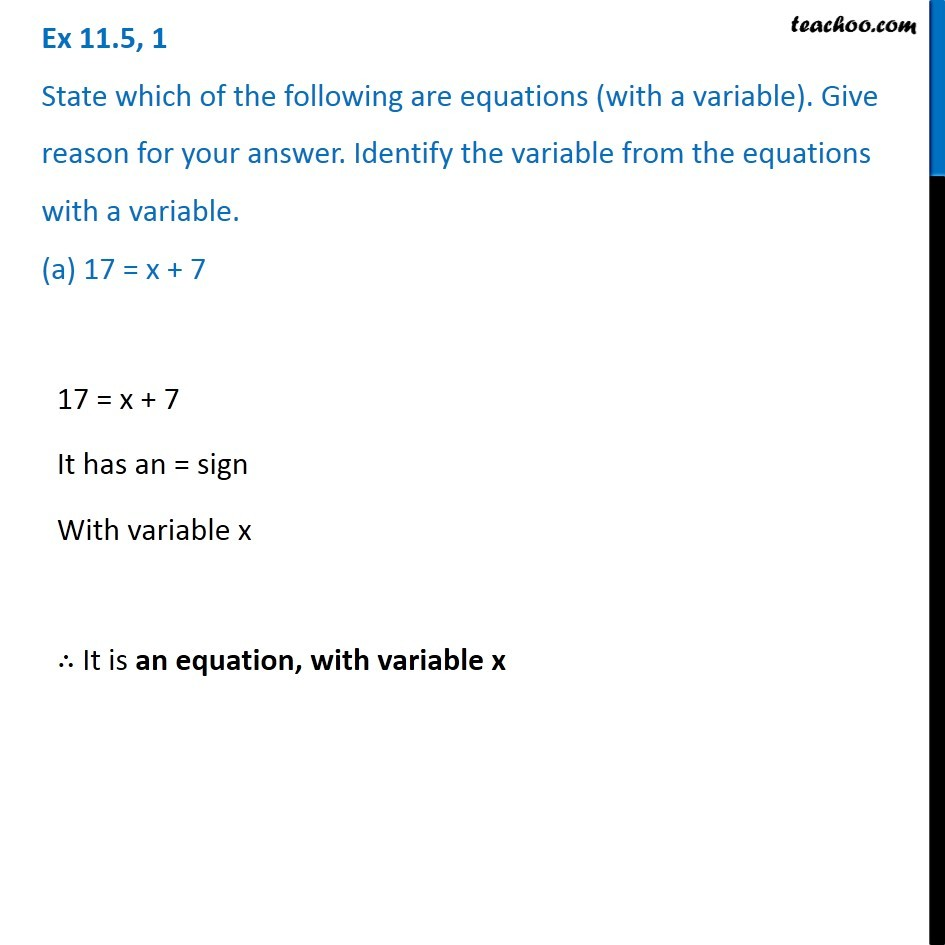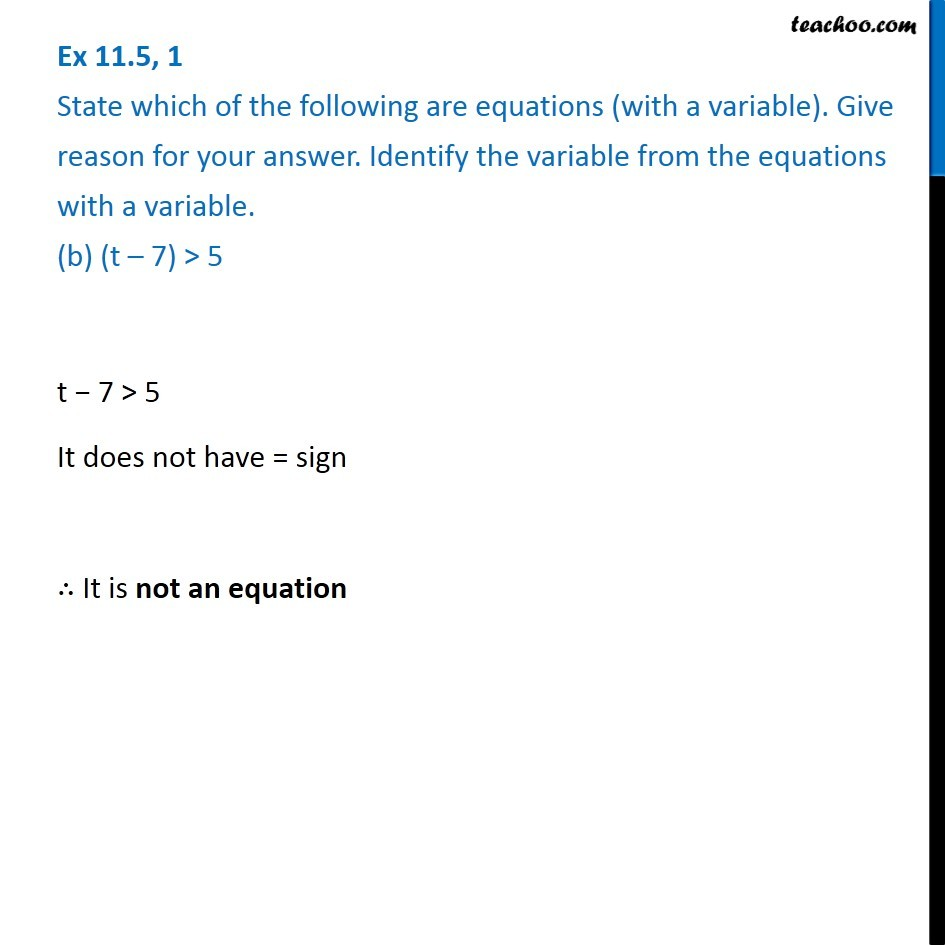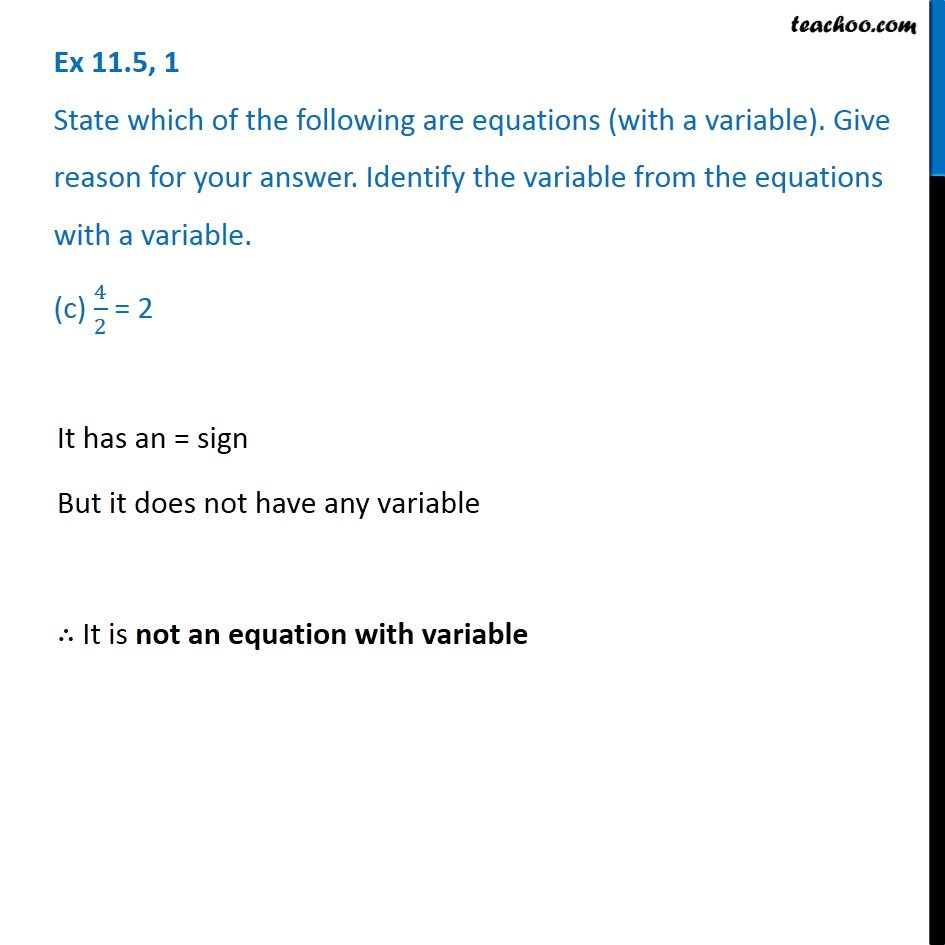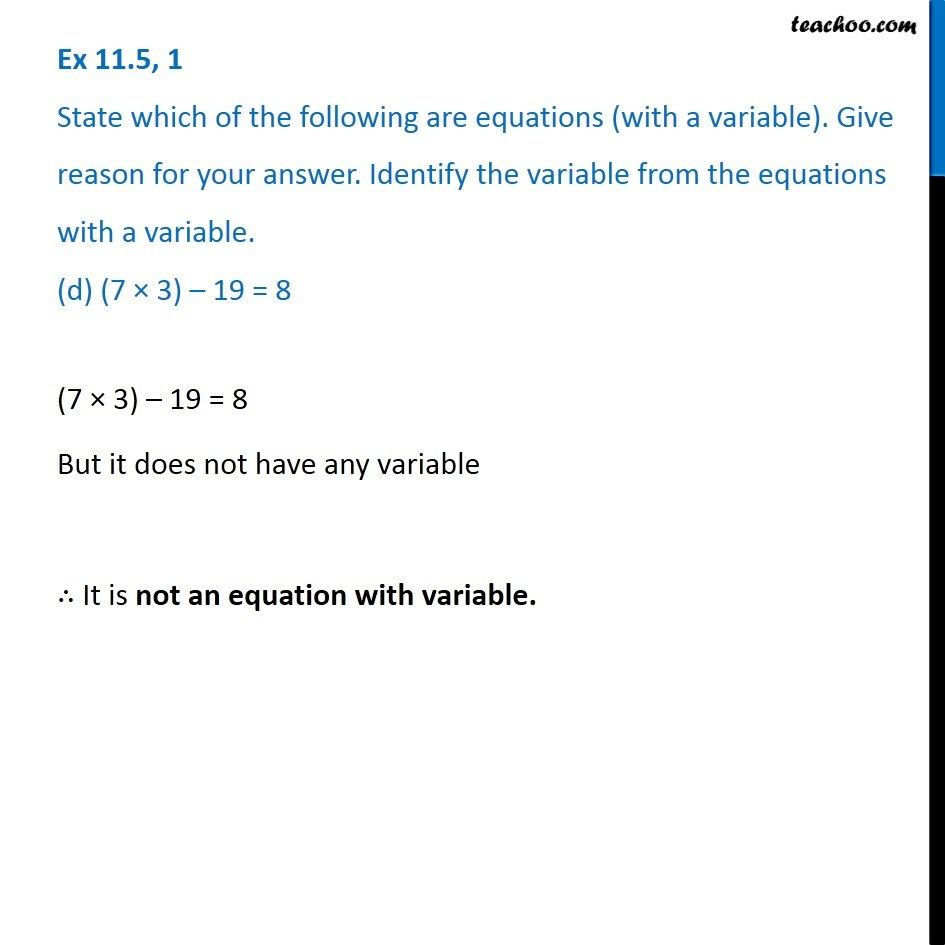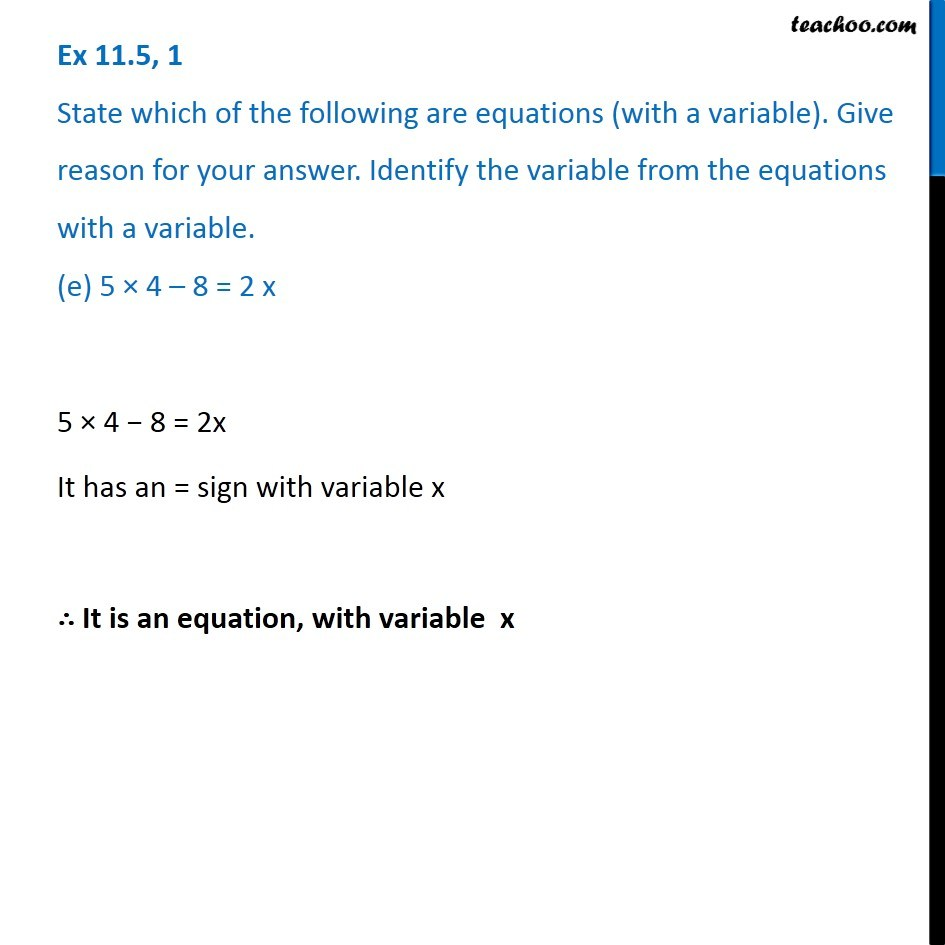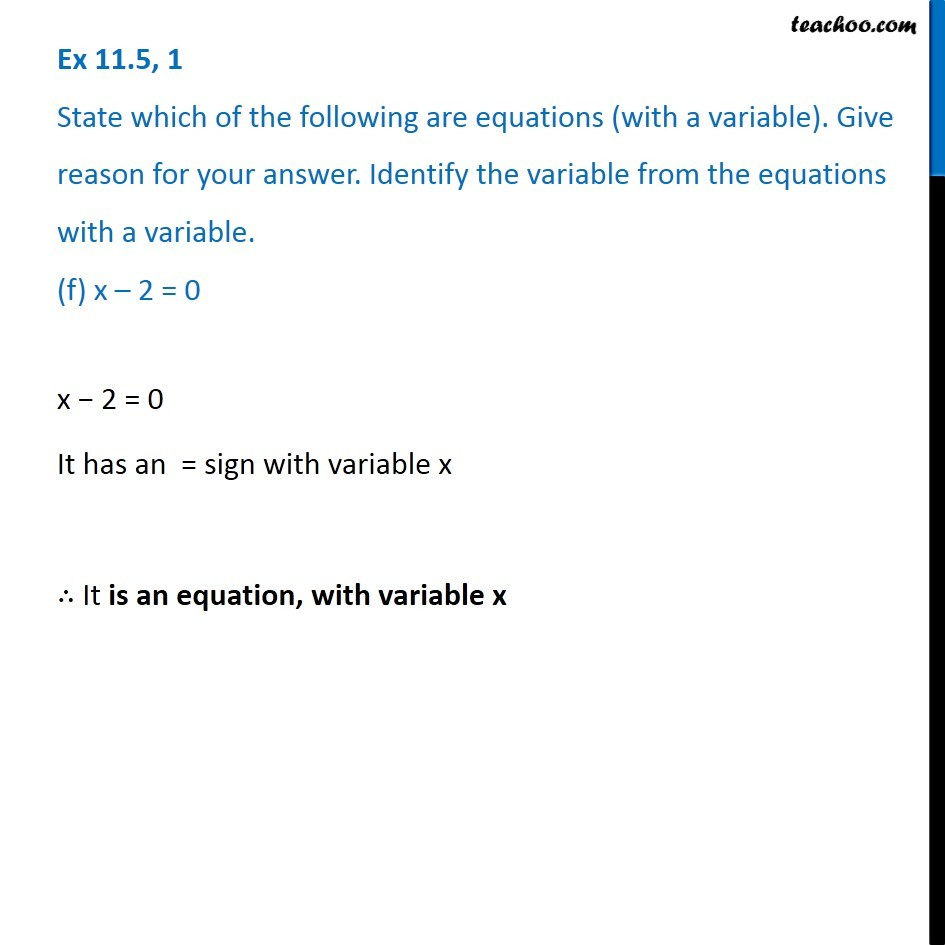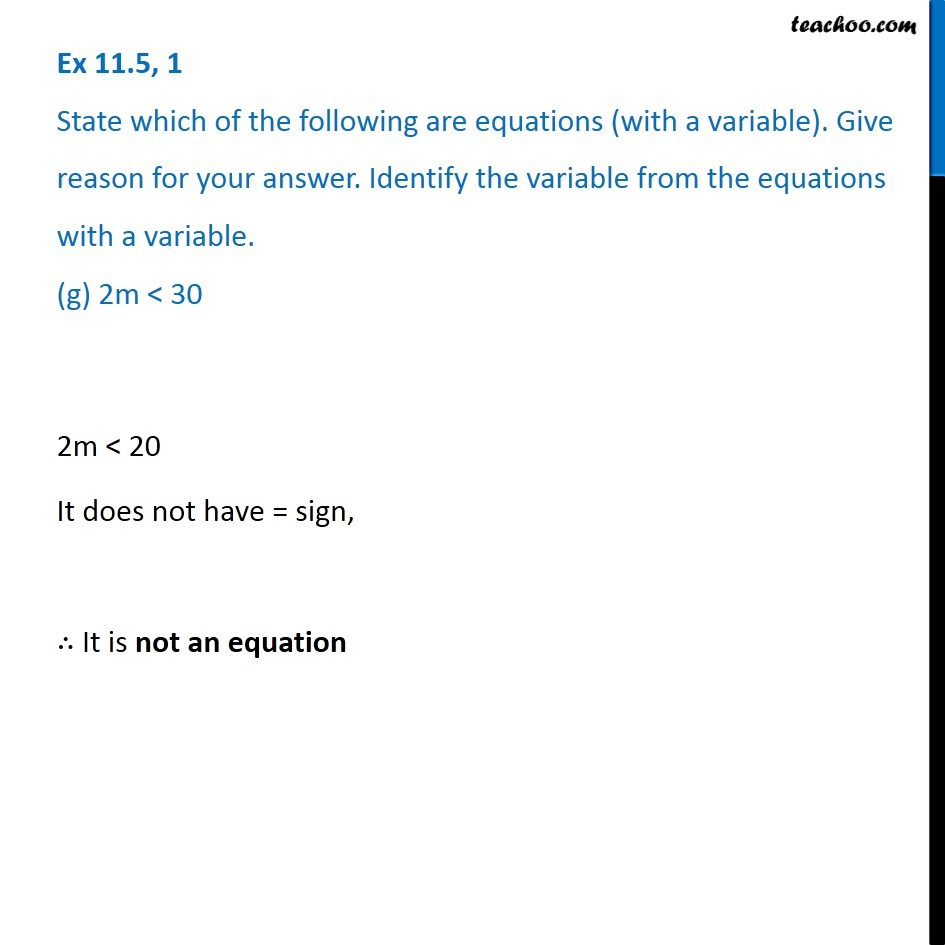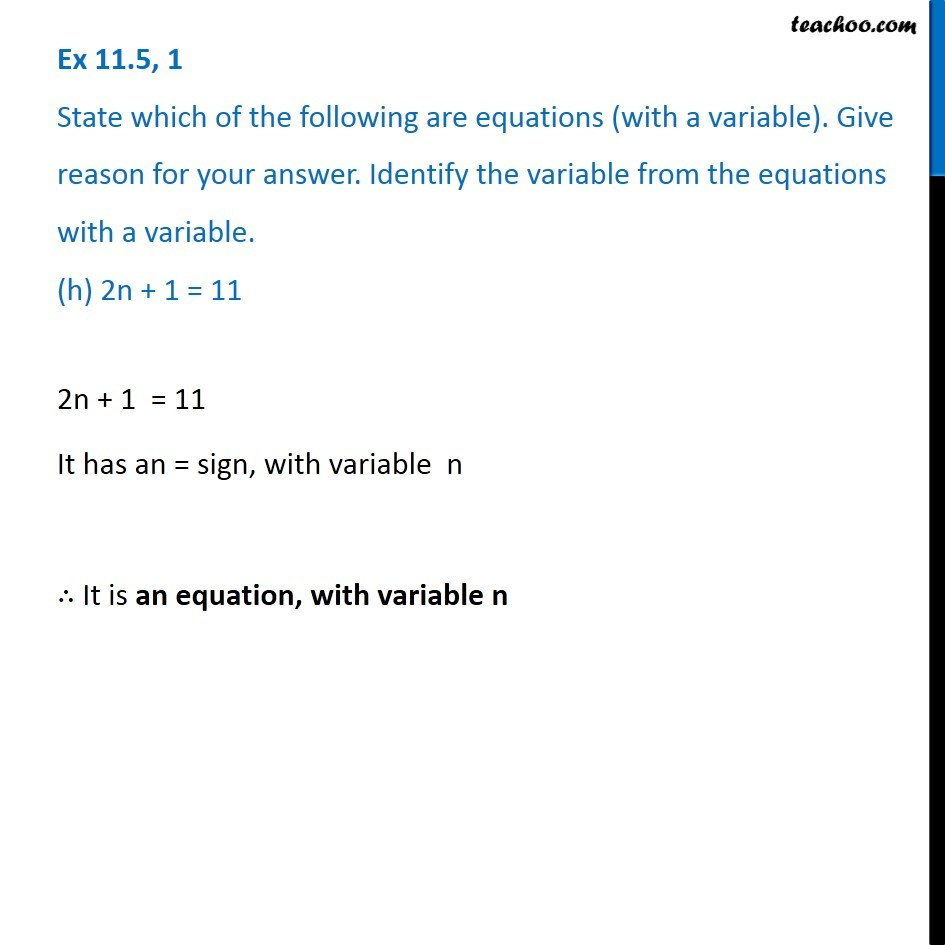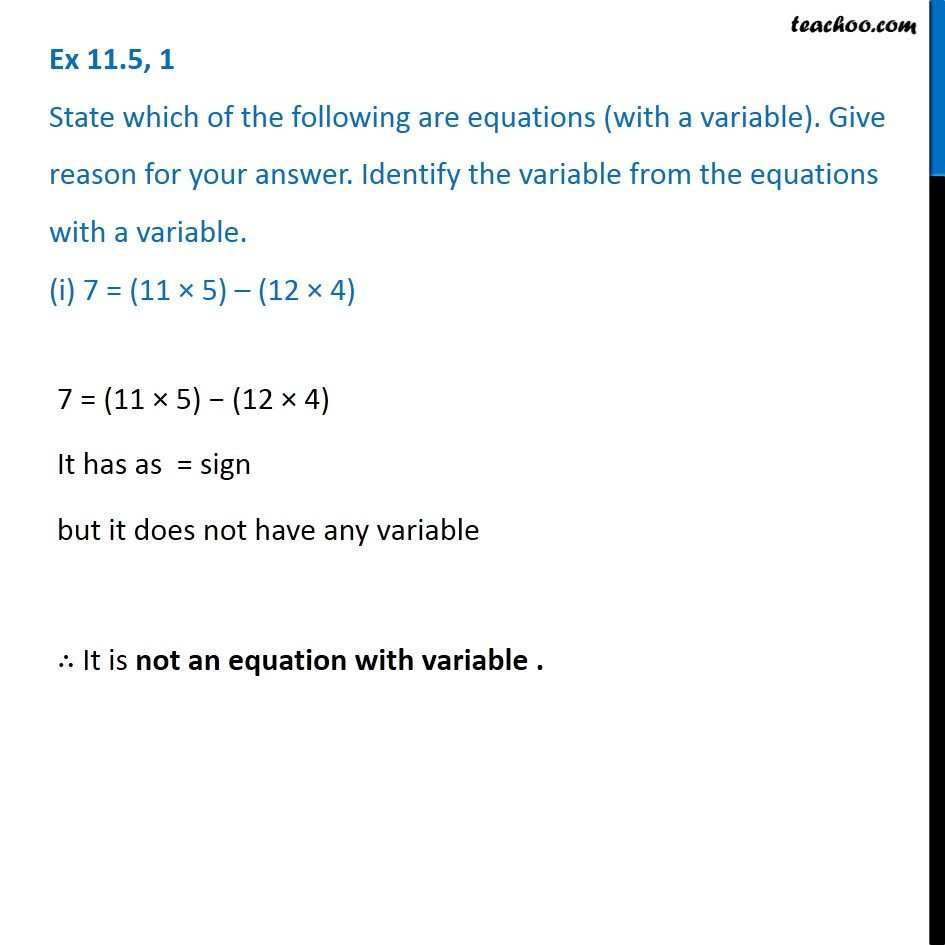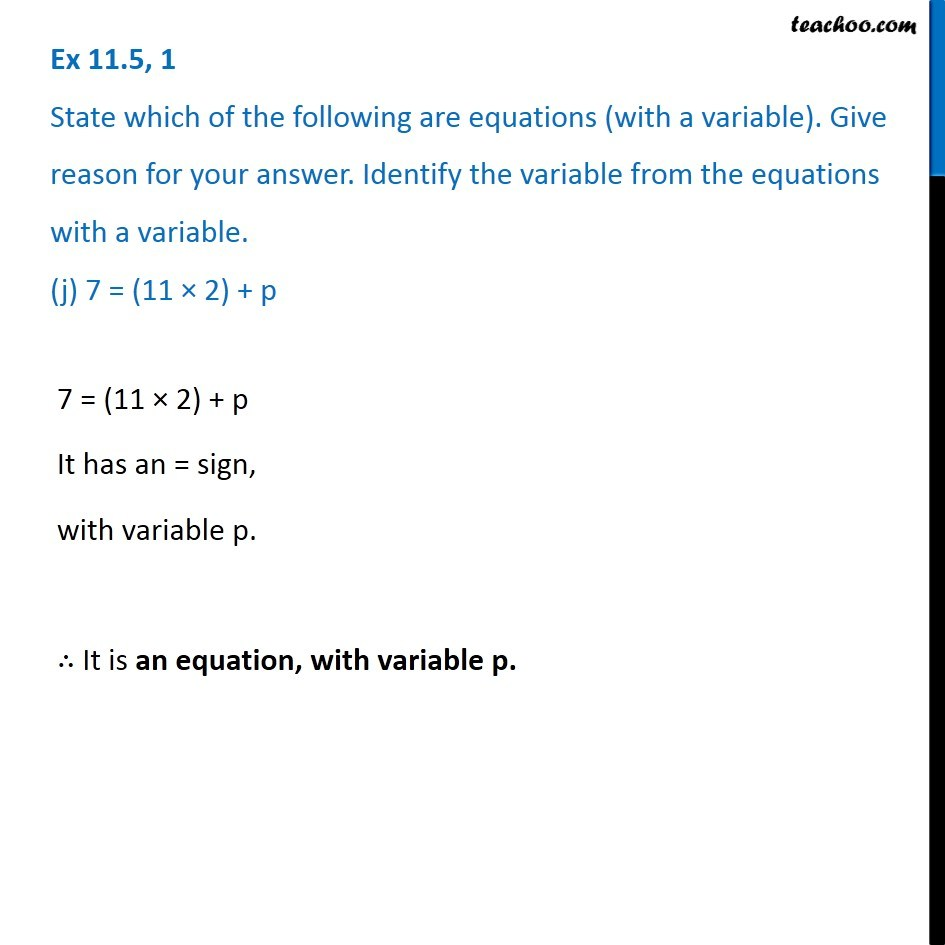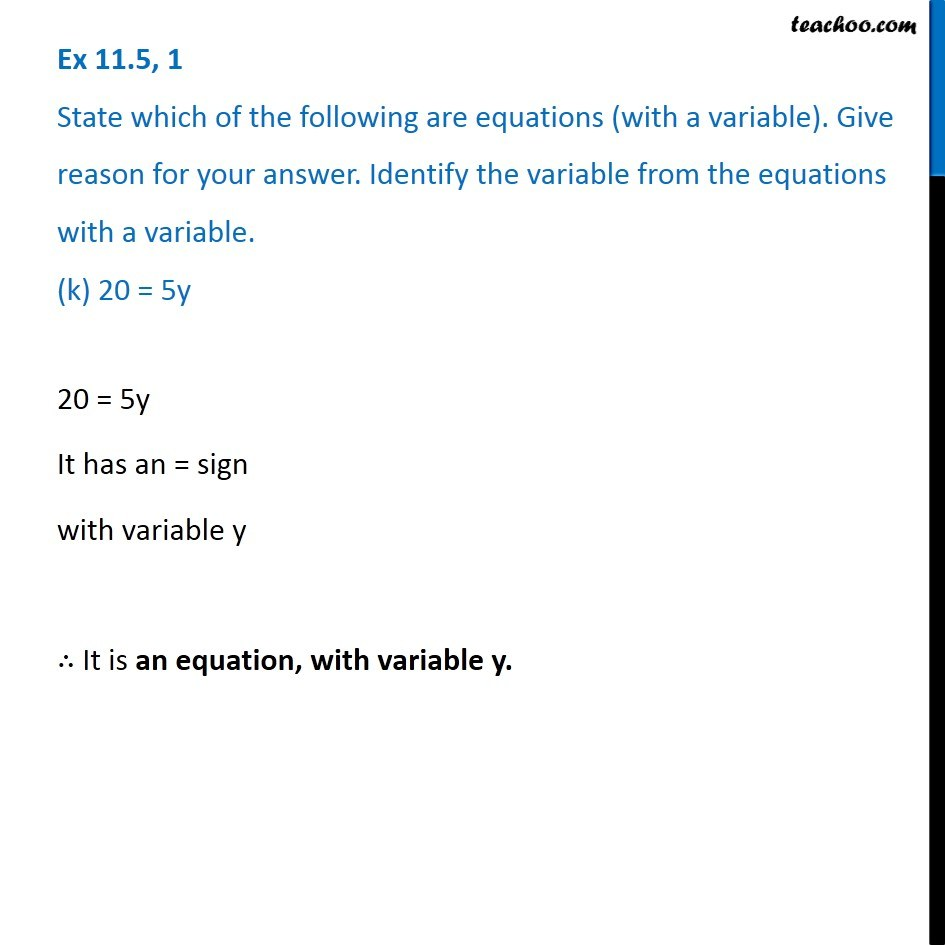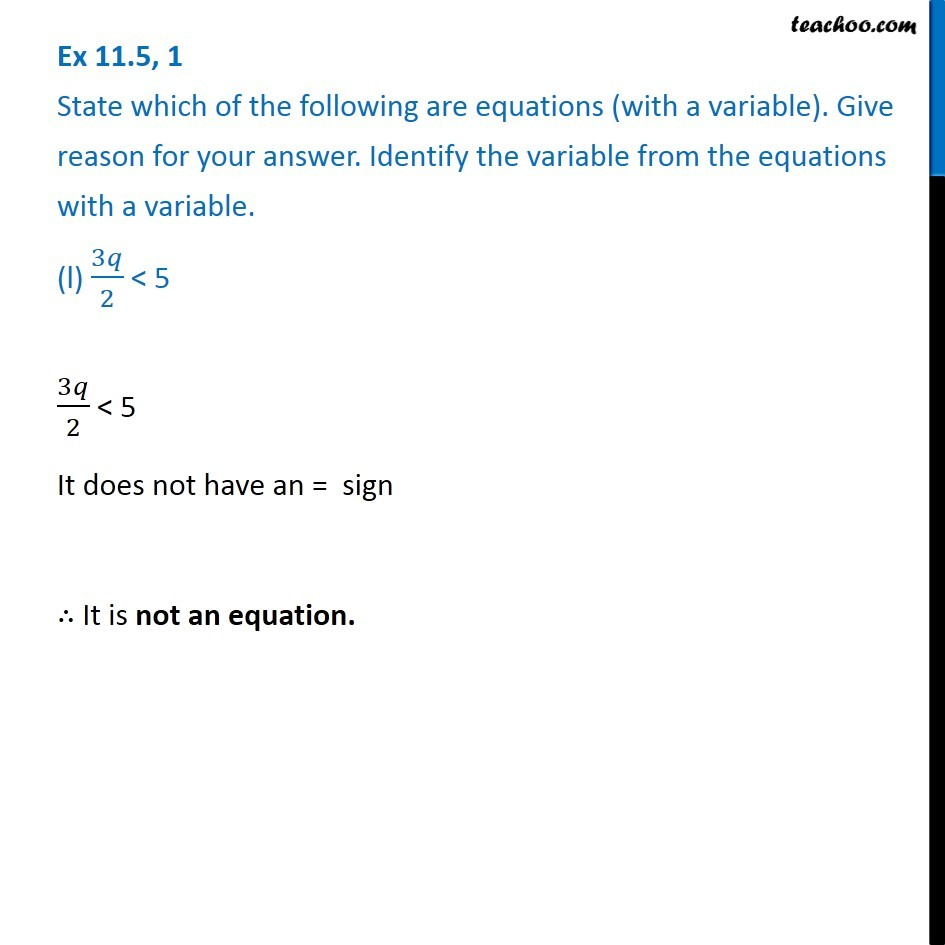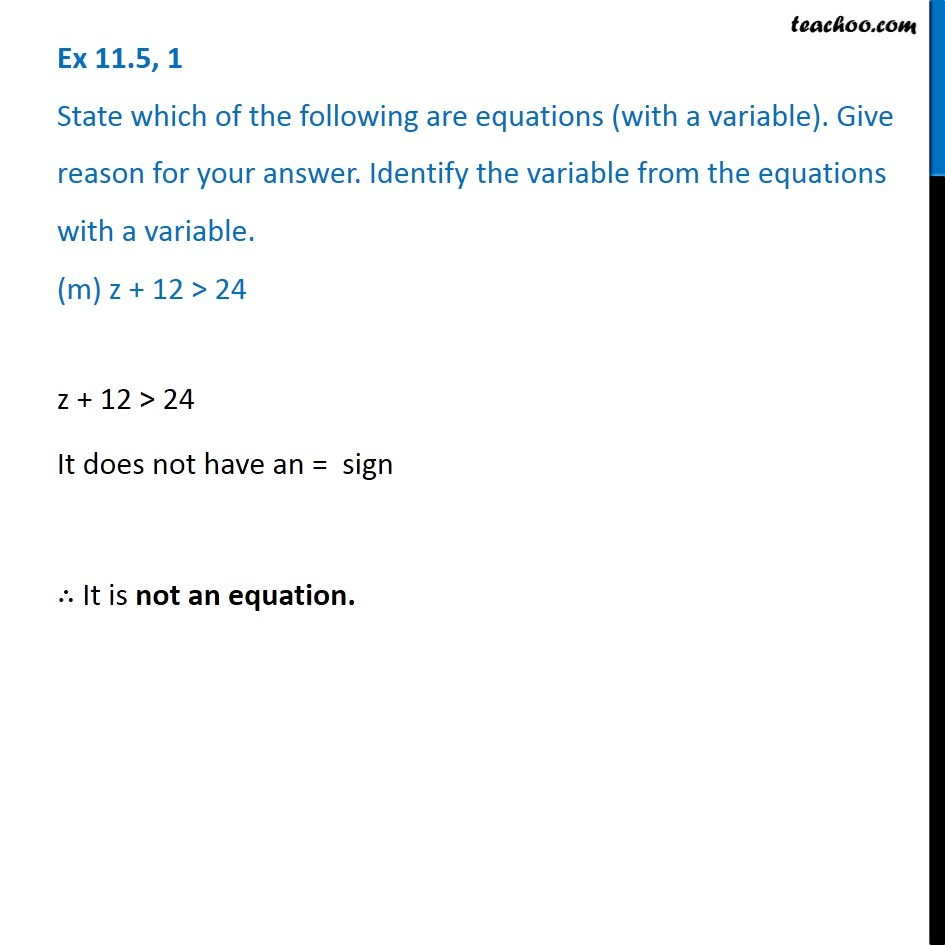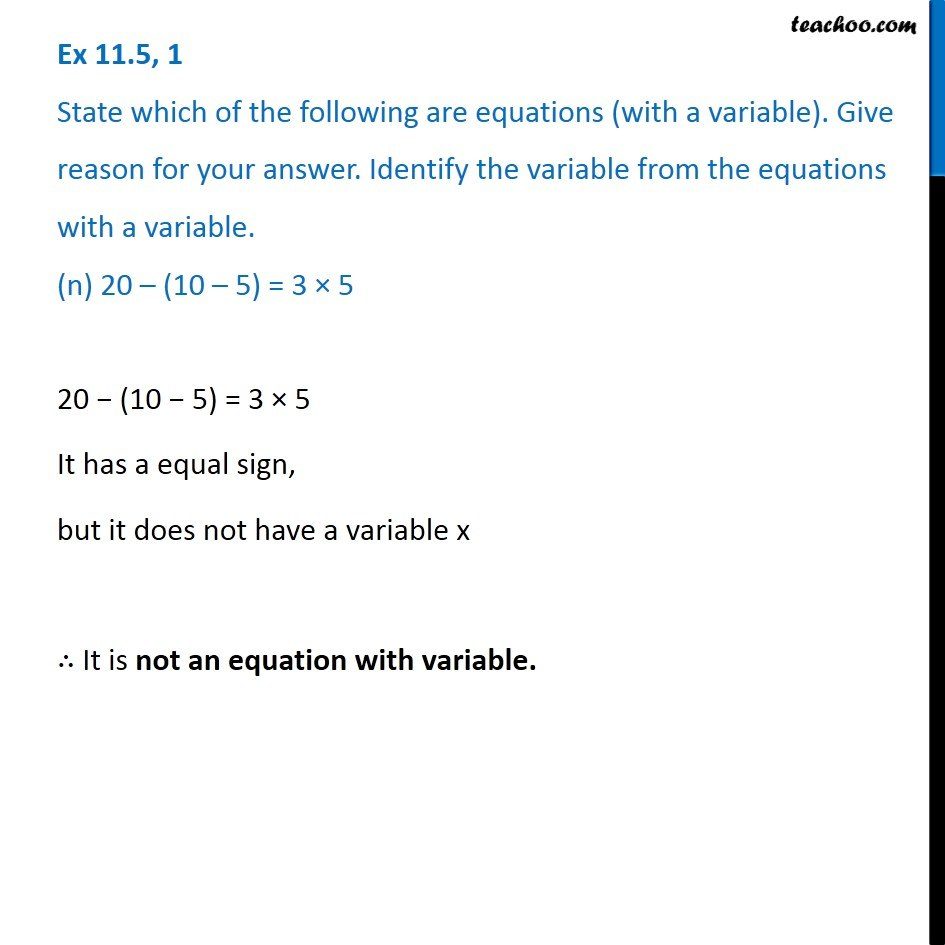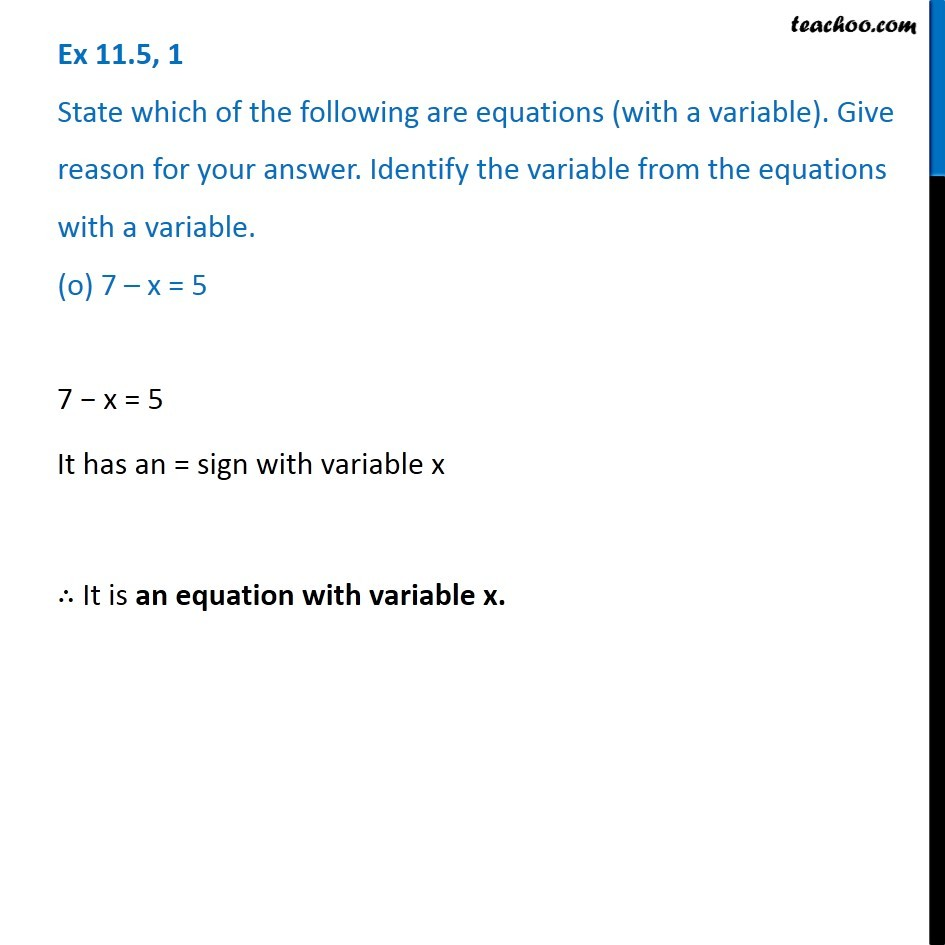1. Chapter 11 Class 6 Algebra
2. Serial order wise
3. Ex 11.5

Transcript

Ex 11.5, 1 State which of the following are equations (with a variable). Give reason for your answer. Identify the variable from the equations with a variable. (a) 17 = x + 717 = x + 7 It has an = sign With variable x ∴ It is an equation, with variable x Ex 11.5, 1 State which of the following are equations (with a variable). Give reason for your answer. Identify the variable from the equations with a variable. (b) (t – 7) > 5t − 7 > 5 It does not have = sign ∴ It is not an equation Ex 11.5, 1 State which of the following are equations (with a variable). Give reason for your answer. Identify the variable from the equations with a variable. (c) 4/2 = 2It has an = sign But it does not have any variable ∴ It is not an equation with variable Ex 11.5, 1 State which of the following are equations (with a variable). Give reason for your answer. Identify the variable from the equations with a variable. (d) (7 × 3) – 19 = 8(7 × 3) – 19 = 8 But it does not have any variable ∴ It is not an equation with variable. Ex 11.5, 1 State which of the following are equations (with a variable). Give reason for your answer. Identify the variable from the equations with a variable. (e) 5 × 4 – 8 = 2 x5 × 4 − 8 = 2x It has an = sign with variable x ∴ It is an equation, with variable x Ex 11.5, 1 State which of the following are equations (with a variable). Give reason for your answer. Identify the variable from the equations with a variable. (f) x – 2 = 0Ex 11.5, 1 State which of the following are equations (with a variable). Give reason for your answer. Identify the variable from the equations with a variable. (f) x – 2 = 0Ex 11.5, 1 State which of the following are equations (with a variable). Give reason for your answer. Identify the variable from the equations with a variable. (g) 2m < 30 2m < 20 It does not have = sign, ∴ It is not an equation Ex 11.5, 1 State which of the following are equations (with a variable). Give reason for your answer. Identify the variable from the equations with a variable. (h) 2n + 1 = 112n + 1 = 11 It has an = sign, with variable n ∴ It is an equation, with variable n Ex 11.5, 1 State which of the following are equations (with a variable). Give reason for your answer. Identify the variable from the equations with a variable. (i) 7 = (11 × 5) – (12 × 4)7 = (11 × 5) − (12 × 4) It has as = sign but it does not have any variable ∴ It is not an equation with variable . Ex 11.5, 1 State which of the following are equations (with a variable). Give reason for your answer. Identify the variable from the equations with a variable. (j) 7 = (11 × 2) + pEx 11.5, 1 State which of the following are equations (with a variable). Give reason for your answer. Identify the variable from the equations with a variable. (j) 7 = (11 × 2) + p Ex 11.5, 1 State which of the following are equations (with a variable). Give reason for your answer. Identify the variable from the equations with a variable. (k) 20 = 5y20 = 5y It has an = sign with variable y ∴ It is an equation, with variable y. Ex 11.5, 1 State which of the following are equations (with a variable). Give reason for your answer. Identify the variable from the equations with a variable. (l) 3𝑞/2 < 53𝑞/2 < 5 It does not have an = sign ∴ It is not an equation. 3𝑞/2 < 5 It does not have an = sign ∴ It is not an equation. z + 12 > 24 It does not have an = sign ∴ It is not an equation. Ex 11.5, 1 State which of the following are equations (with a variable). Give reason for your answer. Identify the variable from the equations with a variable. (n) 20 – (10 – 5) = 3 × 5Ex 11.5, 1 State which of the following are equations (with a variable). Give reason for your answer. Identify the variable from the equations with a variable. (n) 20 – (10 – 5) = 3 × 5Ex 11.5, 1 State which of the following are equations (with a variable). Give reason for your answer. Identify the variable from the equations with a variable. (o) 7 – x = 57 − x = 5 It has an = sign with variable x ∴ It is an equation with variable x.

Ex 11.5

Chapter 11 Class 6 Algebra
Serial order wise

About the AuthorDavneet Singh
Davneet Singh is a graduate from Indian Institute of Technology, Kanpur. He has been teaching from the past 9 years. He provides courses for Maths and Science at Teachoo.